GFG App
Open AppBrowser
Continue

# How to Add and Use an Excel Pivot Table Calculated Field?

Excel pivot tables are one of its most helpful features. They are utilized to summarize or aggregate large quantities of data. The data can be summarized using the average, the count, or other statistical approaches. It summarizes a large amount of data into a few rows and columns. They make it simple to explore the data from various views and angles and are excellent for data exploration.

When you create a Pivot table, you often need to add more data and calculations to your analysis. If you require a new data point that can be obtained by leveraging existing data points in the Pivot Table, you don’t need to go back and add it to the source data. Instead, you can utilize a Pivot Table Calculated Field to accomplish this.

## Pivot Table Calculated Field

The “Pivot Table calculated field” option allows you to insert more data or calculations in the pivot table. For our reports, we frequently need to include our own unique calculations that can relate to other fields in the data collection.

### Benefits of using a Pivot Table Calculated Field

1. It is scalable since it will automatically account for any new data added to your Pivot Table. You can utilize a Calculate Field in your Pivot Table just like any other field.
2. It does not necessitate the use of formulas or the updating of source data.
3. Easy to update and manage. If the metrics or computation changes, you can do it via the Pivot Table.

### Adding a Calculated Field to the Pivot Table

The following are the steps for adding a Pivot Table Calculated Field:

Step 1: Select any cell in the pivot table in your spreadsheet and Click on Analyze from the top ribbon.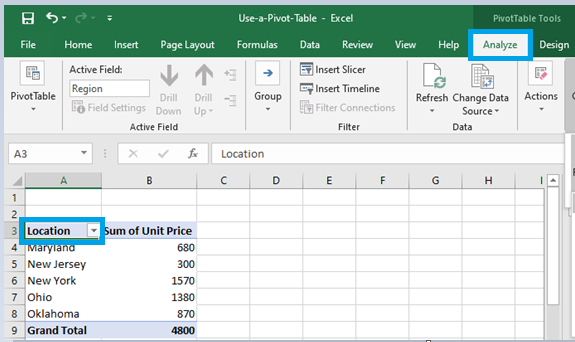Step 2: Navigate to the Calculations tab.Step 3: Select the option Fields, Items, & Sets.Adding and Using an Excel Pivot Table Calculated Field

Step 4: Click on Calculated Field from the drop-down menu.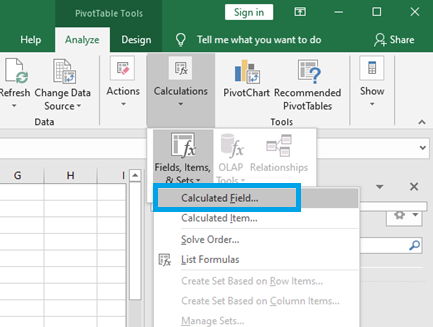The dialog box Insert Calculated Field appears. This is where you will name and create the field.

Step 5: Enter a name and Formula for the calculated field in the Name and Formula fields respectively.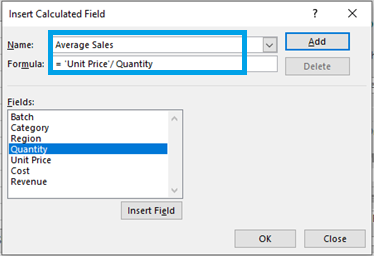Note: Formulas can include standard operational signs and built-in program functions, however, they are incompatible with cell references and array formulas. Select the name of a field from the “Fields” box and click “Insert Field” to use it in your formula.

Step 6: Click on Add, the Calculated Field will appear as one of the fields in the PivotTable Fields list as soon as you add it, then Click on OK: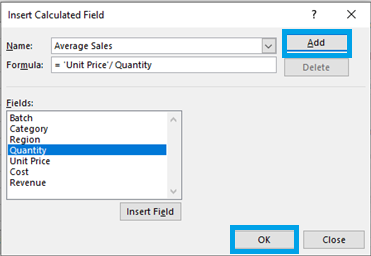### How to Modify or Delete a Pivot Table Calculated Field?

After you’ve created a Pivot Table Calculated Field, you can modify or delete the formula by following these steps:

Step 1: Select any cell in the pivot table in your spreadsheet and Click on Analyze from the top ribbon.Step 2: Navigate to the Calculations tab.Step 3: Select the option Fields, Items, & Sets.Step 4: Click on Calculated Field from the drop-down menu.Step 5: Click the drop-down arrow in the Name field (small downward arrow at the end of the field).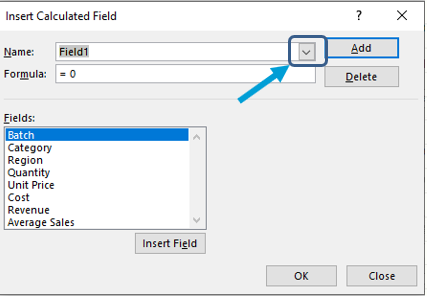Step 6: Select the calculated field you want to delete or modify from the list.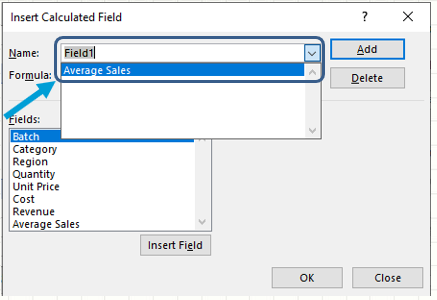Step 7: If you wish to modify the formula, change it, or click Delete if you want to remove it.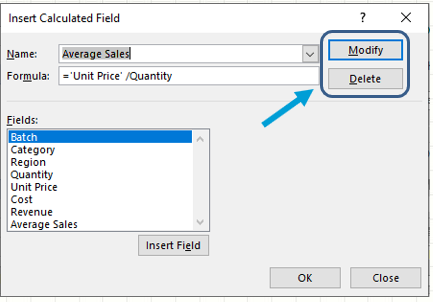### How to Get a List of All the Calculated Field Formulas?

Excel makes it simple to generate a list of all the formulas used in the creation of Calculated Fields. The following are the procedures to easily obtain a list of All Calculated Fields formulas:

Step 1: Select any cell in the pivot table in your spreadsheet and Click on Analyze from the top ribbon.Step 2: Navigate to the Calculations tab.Step 3: Select the option Fields, Items, & Sets.Step 4: Click on Calculated Field from the drop-down menu.Step 5: Click on List Formulas.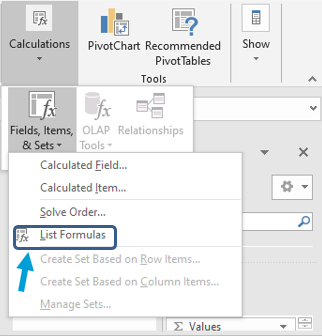Step 6: When you select List Formulas, Excel will automatically create a new worksheet with information about all the calculated fields and items you have used in the pivot table.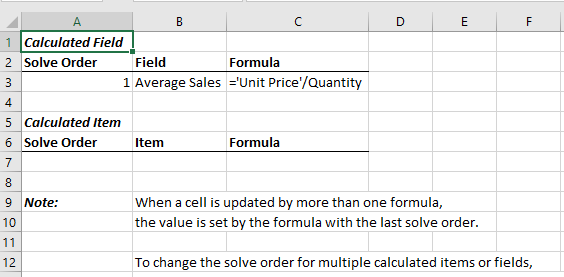My Personal Notes arrow_drop_up